# Finding the Derivative of the Square Root of x

An error occurred trying to load this video.

Try refreshing the page, or contact customer support.

Coming up next: Solving the Derivative of cos(2x)

### You're on a roll. Keep up the good work!

Replay
Your next lesson will play in 10 seconds
• 0:04 Steps to Solve
• 1:05 Solution
• 1:35 Checking Your Work
• 4:30 Integrals

Want to watch this again later?

Timeline
Autoplay
Autoplay
Speed

#### Recommended Lessons and Courses for You

Lesson Transcript
Instructor: Laura Pennington

Laura has taught collegiate mathematics and holds a master's degree in pure mathematics.

This lesson will show how to find the derivative of the square root of x. We will then look at two different ways to check our work: one involving limits and the other involving integrals.

## Steps to Solve

We want to find the derivative of the square root of x. To get started, we need to be aware that the square root of x is the same as x raised to the power of 1/2. In general, we know that the nth root of x is equal to x raised to the power of 1/n. Since the square root of x is the second root of x, it is equal to x raised to the power of 1/2.

You may be wondering why we want to think of the square root of x in this way. Well, as it turns out, we have a nice formula we can use to find the derivative of xa.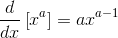Thus, if we think of the square root of x as x 1/2, then we can use the formula to find the derivative.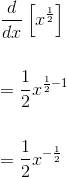## Solution

The formula gives that the derivative of the square root of x is (1/2)x -1/2. This can be written in a few different forms: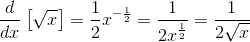## Checking Your Work

There are a couple different ways that we can check our work when dealing with derivatives. The first deals with the definition of a derivative using limits.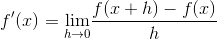We can use this definition to check our work. In doing so, we should get the same result as we did when using the formula. We start by letting f(x) = sqrt(x), and we plug in accordingly.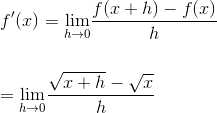Now we want to find the limit as h approaches 0. One way of evaluating a limit is by plugging the number that h is approaching in for h. However, in this case, we would be plugging in 0 for h. Can you see why we can't do that? If you are thinking that we can't plug in 0 for h because that would create a zero denominator, then you are correct! Therefore, we are going to manipulate the limit to get it into a form where we can plug in 0 for h without creating an undefined expression. We will multiply it all by a version of the number 1:Remember, we didn't change the limit since we ultimately just multiplied it by one. Also, notice that we now are able to plug in zero for h without creating a zero denominator or an undefined expression. Let's do just that to find the limit and, in the process, find the derivative of the square root of x. Once we plug 0 in for h, our equation becomes: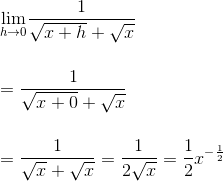To unlock this lesson you must be a Study.com Member.

### Register to view this lesson

Are you a student or a teacher?

### Unlock Your Education

#### See for yourself why 30 million people use Study.com

##### Become a Study.com member and start learning now.
Back
What teachers are saying about Study.com

### Earning College Credit

Did you know… We have over 160 college courses that prepare you to earn credit by exam that is accepted by over 1,500 colleges and universities. You can test out of the first two years of college and save thousands off your degree. Anyone can earn credit-by-exam regardless of age or education level.